Economic Dispatch with Emission and Transmission Constraints by Augmented Lagrange Hopfield Network | OMICS International
Global Journal of Technology and Optimization

# Economic Dispatch with Emission and Transmission Constraints by Augmented Lagrange Hopfield Network

Vo Ngoc Dieu, Weerakorn Ongsakul*

Asian Institute of Technology, Klongluang, Pathumthani, Thailand

*Corresponding Author:
Weerakorn Ongsakul
Asian Institute of Technology, Klongluang, Pathumthani, Thailand
E-mail: [email protected]

Received date: May 2009; Revised date: July 2009; Accepted date: October 2009

Visit for more related articles at Global Journal of Technology and Optimization

#### Abstract

This paper proposes an augmented Lagrange Hopfield network (ALHN) for solving economic dispatch (ED) problem with ramp rate, emission and transmission constraints. The proposed ALHN method is the continuous Hopfield neural network with its energy function based on augmented Lagrangian function. In ALHN, the energy function is augmented by Hopfield terms from Hopfield neural network and penalty factors from augmented Lagrangian function to damp out oscillation of the Hopfield network during its convergence. Consequently, ALHN can overcome the drawbacks of the conventional Hopfield network due to its simplicity, better optimal solution and faster computing time. The proposed method has been tested on large-scale systems up to 1,200 units and the New England 39-bus system and the obtained results are compared to other methods available in the literature. The test results have shown that the proposed method is more favorable than the others for less total costs and faster computational times.

#### Keywords

Augmented Lagrange Hopfield network, economic dispatch, emission constraint, transmission constraint.

Nomenclature

ai, bi, ci Fuel cost coefficients for unit i

Bij Transmission loss formula coefficient

Dli Generalized generation distribution factor for calculating power flow on transmission line l due to generator i

DRi Ramp down rate limit of unit i (MW/h)

EMk System mission allowance during interval k (ton/h)

ERi Emission rate of unit i (kg/MWh)

Err(n) max Maximum error of neural network at iteration n

FCi Cost of the fuel consumed by unit i (\$/MWh)

M Number of subintervals during schedule time horizon

N Total number of online units

NL Number of transmission lines

Nmax Maximum number of iterations of ALHN

PDk Total load demand of the system during interval k (MW)

Pi,min Lower generation limit of unit i (MW)

Pi,max Upper generation limit of unit i (MW)

Pik Output power of unit i at interval k (MW)

Pik,high Maximal possible power output of unit i during interval k (MW)

Pik,low Minimal possible power output of unit i during interval k (MW)

Pik-1 Output power of unit i at interval k-1 (MW)

Pl,max Maximum power flow on transmission line l (MW)

Plk Power low on transmission line l at interval k (MW)

PLk Total network loss of the system at interval k (MW)

sk Sign function for emission constraint in Lagrangian relaxation handling inequality constraint

Uλk Input of multiplier neuron corresponding to the output Vλk

Uγk Input of multiplier neuron corresponding to the output Vγk

Uik,p Input of continuous neuron corresponding to the output Vik,p

Ulk Input of multiplier neuron corresponding to the output Vlk

Ulk,p Input of continuous neuron corresponding to the output Vlk,p

URi Ramp up rate limit of unit i (MW/h)

Vλk Output of multiplier neuron representing λk

Vγk Output of multiplier neuron representing γk

Vik,p Output of continuous neuron representing Pik

Vlk Output of multiplier neuron representing ηlk

Vlk,p Output of continuous neuron representing Plk,p

σ Slope of sigmoid function of continuous neurons

βγ Penalty factor associated with emission constraint

βλ Penalty factor associated with power balance

βη Penalty factor associated with transmission constraint

ε Maximum tolerance for the neural network

αλ, αγ, αη Updating step sizes for multiplier neurons

αil Updating step sizes for continuous neurons

κi Ratio between emission rate and fuel cost for unit i

γk Lagrangian multiplier associated with emission constraint at subinterval k

λk Lagrangian multiplier associated with power balance constraint at subinterval k

ηlk Lagrangian multiplier associated with transmission constraint l at subinterval k

#### Introduction

In optimal power generation, the economic dispatch (ED) problem has been extensively studied due to its benefit in economic generation operation. The objective of the ED problem is to determine the amount of real power contributed by online thermal generators satisfying load demand at any time subject to unit and system constraints so as the total generation cost is minimized. Therefore, it is very important to solve the problem as quickly and precisely as possible.

Several conventional methods have been applied for solving ED problem such as linear programming (LP) , lambda iteration, gradient search, Newton’s method, dynamic programming  and Lagrangian relaxation . The conventional methods can find good solutions in a fast manner. However, they can only be applied to small-scale and simple problems. Recently, many techniques based on artificial intelligence have been also used for solving ED problem including genetic algorithm (GA) , tabu search (TS) , simulated annealing (SA) , evolutionary programming (EP) , ant colony search algorithm (ACSA) , particle swarm optimization (PSO) , and Hopfield neural networks (HNN) [10,11]. These methods can deal with more complicated problems than the classical methods. However, they still suffer slow convergence rate to the near optimal solutions. Therefore, they need more improvements to obtain better than solutions. Consequently, hybrid systems are one of the new trends for solving optimization problems. Hybrid systems have been applied for solving ED problem such as hybrid method based on evolution differential  and hybrid Hopfield network and QP (Hybrid HNN-QP) . These hybrid methods are more powerful than the single methods due to their ability to deal with complicated problems and obtain better optimal solution and faster computational time.

In this paper, an augmented Lagrange Hopfield network (ALHN) is proposed for solving ED problem with ramp rate, emission and transmission constraints. The proposed ALHN method is the continuous Hopfield neural network with its energy function based on augmented Lagrangian function. In ALHN, the energy function is augmented by Hopfield terms from Hopfield neural network and penalty factors from augmented Lagrangian function to damp out oscillation of the Hopfield network during its convergence. Consequently, ALHN can overcome the drawbacks of the conventional Hopfield network due to its simplicity, better optimal solution and faster computing time. The proposed method has been tested on large-scale systems up to 1,200 units and the New England 39-bus system and the obtained results are compared to other methods available in the literature including enhanced Hopfield neural network (EHNN) , SA, GA, hybrid differential evolution (HDE), variable scaling HDE (VSHDE) , improved Hopfield neural network (IHNN) , LP , QP and SQP.

#### Problem formulation

The objective of the ED problem with emission and transmission constraints is to minimize total cost of thermal generating units of a system over some appropriate periods (one hour typically) while satisfying various constraints including power balance, generator power limits, ramp rate, emission and transmission constraints.

Mathematically, the problem is formulated as follows: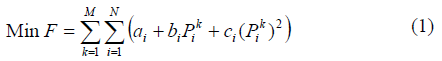subject to

(a) Power balance constraint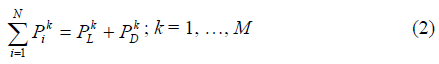where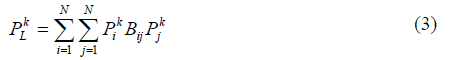(b) Generator operating limits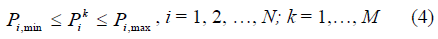(c) Ramp rate constraints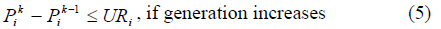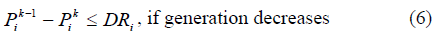(d) Emission limitation constraint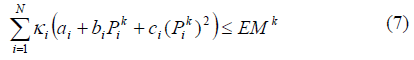where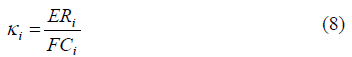(e) Transmission constraint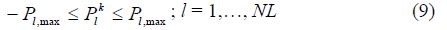where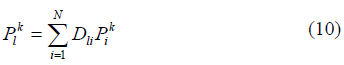#### An Augmented Lagrange Hopfield network

For the implementation of the ALHN, the augmented Lagrangian function for the problem is firstly formulated, and then energy function of the ALHN is constructed based on the founded augmented Lagrangian function with augmentation of Hopfield terms. In the proposed ALHN, equality and inequality constraints can be easily handled by augmented Lagrangian function while variables limits are efficiently handled by sigmoid function from Hopfield network.

Calculation of generalized generation distribution factor

In this paper, the power flow in transmission lines is computed using DC power load flow method which focuses only on real power flow supposing that the voltage at each bus is equal to 1 pu and resistance of transmission lines is negligible. The generalized generation distribution factor (GGDF)  is used for calculating power flow in transmission constraint based on generation shift distribution factor (GSDF) which is based on DC power flow . The GGDF method is used for directly calculating DC power flow in transmission lines without running load flow. Using GSDF, the total system generation remains unchanged since all the generation shifts are absorbed by the reference bus. If load demand changes, a new load flow will be run to re-establish the initial values since the initial line flows must be known in advance to calculate power flows in transmission lines based on GSDF. The advantage of GGDF over GSDF is that it is not necessary to be recalculated when load demand changes. Therefore, GGDF is more suitable for DC power flow calculation in transmission constraint of the ED problem.

The GGDF coefficient Dln representing power on line l due to power generation at bus n is derived as follows :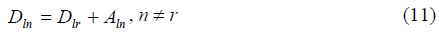where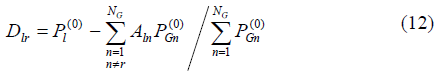and r is reference bus of the system, Aln is GSDF representing power flow on line l due to power generation at bus n, Dlr is GGDF representing power flow on line l due to power generation at reference bus, and power flow Pl (0) on line l is calculated corresponding to total generation PGn(0) at each bus n by DC power flow method .

Calculation of B-coefficients

The B-coefficients method is an approximate method widely used for calculating power loss in transmission lines in ED problems. The B-coefficients can be derived out in terms of GGDF under the DC approximation as follows .

The total power loss of the system is expressed in terms of resistance and power flows in transmission lines: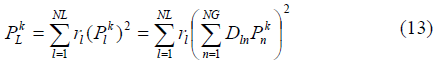where Pnk is power generation at bus n at during interval k, NG is the number of generation buses, and rl the is resistance of transmission line l.

On the other hand, the total power loss is also expressed in terms of B-coefficients as follows: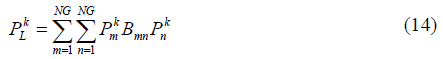From (13) and (14), the B-coefficients are derived out by getting second derivative of total power loss as follows: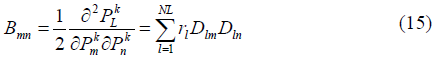The B-coefficients are used for calculating power loss in power balance constraint while the DC load flows are calculated for checking line overload in transmission constraint. Therefore, the B-coefficients are needed even though the line flows are calculated.

Augmented Lagrange Hopfield network

In this ED problem, variables will be considered including power generation Pik and power flow on transmission lines Plk for each subinterval k satisfying their limits, power balance equality constraint, ramp rates and emission inequality constraints. For implementation in ALHN, (N+NL)×M continuous neurons representing continuous variables and (2+NL)×M multiplier neurons representing Lagrangian multipliers are used.

The augmented Lagrange function L is first formulated as follows: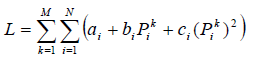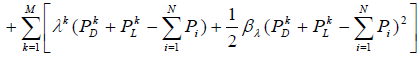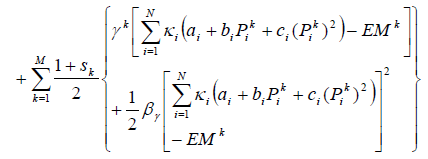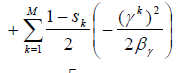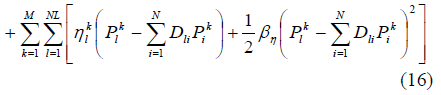where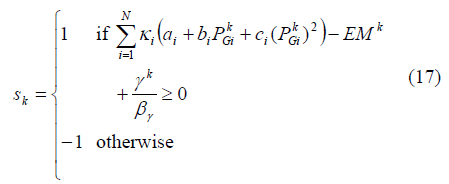In the Lagrangian relaxation method, the inequality constraint of emission is treated in two smooth terms. The derivation and continuity of these terms in Lagrangian relaxation for the inequality constraint are given in Appendix from .

The augmented Lagrange function (16) is converted to the energy function E of ALHN in terms of neurons as follows: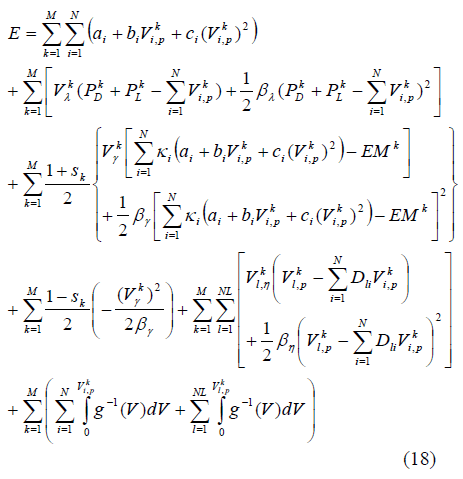In (18), the sums of integral terms are the Hopfield terms where their global effect is a displacement of solutions toward the interior of the state space .

The dynamics of neurons based on the derivative of the energy function with respect to outputs of neurons are determined: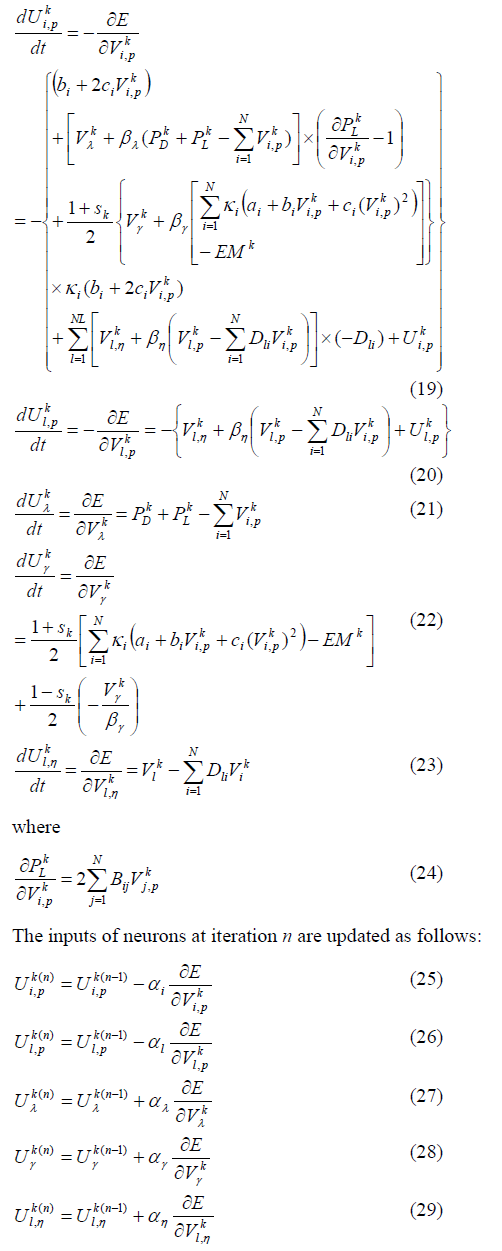The outputs of continuous neurons are determined based on the sigmoid function :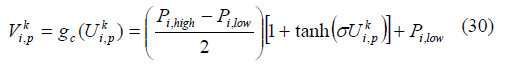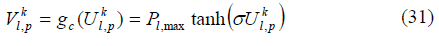where the new generators limits are determined by: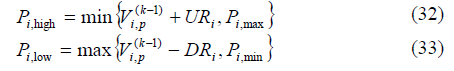The outputs of multiplier neurons are equally set to their inputs by using the linear transfer function. The proof of convergence of the proposed ALHN is given in .

Selection of parameters: In the ALHN model, the parameters have to be predetermined including sigmoid function slope, updating step sizes for neurons and penalty factors for augmented Lagrange function. A proper parameter selection will guarantee rapid convergence to ALHN. The parameters of ALHN are selected via tuning. By experiments, the values of sigmoid function slope and penalty factors are fixed at 100 and 0.001, respectively. The values of the others will vary depending on the data of test systems. It is observed that the larger the value of updating step sizes the closer the discrete system behavior, producing values at the upper and lower limits of each neuron. On the contrary, the smaller the value of updating step sizes the slower convergence of the network.

Initialization: The algorithm requires initial conditions for all neurons. In this paper, the initial outputs of neurons are selected as follows.

For the continuous neurons representing output power of units, their outputs Vi,pk(0) are initiated by “mean distribution” :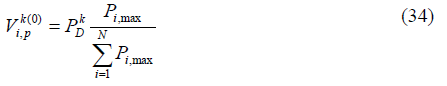For the multiplier neurons associated with power balance constraint, their outputs Vλk (0) are initialized by mean value: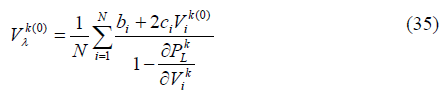The initial values of outputs for other continuous and multiplier neurons are set to zero. The initial inputs of continuous and multiplier neurons are calculated via the inversed functions of the sigmoid and transfer functions, respectively.

Termination criteria: In the proposed ALHN, the maximum error is calculated from the constraint errors and neural iterative errors.

The algorithm will be terminated when either the maximum error Errmax is lower than a pre-specified tolerance ε or maximum number of iterations Nmax is reached.

Overall procedure

The overall procedure of the proposed ALHN for solving ED problem with ramp rate, emission and transmission constraints is as follows:

Step 1: Select sigmoid function slope for continuous neurons, updating step sizes for all neurons and penalty factors as Section 3.3.

Step 2: Initialize outputs of all neurons as Section 3.3 and calculate their corresponding inputs.

Step 3: Set up maximum tolerance and maximum number of iteration for ALHN

Step 4: Set n = 1.

Step 5: Calculate dynamics of neurons using (19)-(23).

Step 6: Update inputs of neurons using (25)-(29).

Step 7: Calculate the corresponding outputs of all neurons using (30)-(31) and linear function.

Step 8: If Err(n) max > ε and n < Nmax, n = n + 1 and return to Step 5. Otherwise, calculate total cost and stop.

Numerical results

The proposed ALHN is tested on various systems and the obtained results are compared to other methods in the literature. Conventional QP and SQP methods have also been implemented to solve the same problems, in which QP is applied for systems with linear constraints while SQP is applied for systems with nonlinear constraints. The algorithms of the proposed method, QP and SQP are implemented on Matlab platform and run on a 1.5 GHz Celeron PC. For stopping criteria, the maximum tolerance of ALHN ε is set to 10-3.

20-unit system

The test system has 20 generating units from  supplying to a load demand of 2,500 MW. The ramp rate, emission and transmission constraints are neglected in this test system. The obtained total cost and computing time from the proposed method are compared to that from EHNN and Newton- Raphson (N-R) method in , and SQP method as shown in Table 1.

The total cost from the proposed method is less than that of NR and EHNN and the same as that of SQP. For the computational time, the proposed method is slightly faster than the others. Note the computer used in  is based on Intel Pentium IV, 1.4-GHz Celeron processor.

40-unit systems

The test system is from practical system of Taiwan power company (TPC) with 40 generating units . In this case, ramp rate, emission and transmission constraints, and power loss are neglected. The proposed method is tested for three load demands of 10,500, 9,500 and 9,000 MW, and the obtained total costs are compared to those from SA, GA, HDE, and VSHDE in , IHNN , and QP method as in Table 2.

In all cases, the proposed method always obtains less total costs than the others except QP. However, the proposed method is faster than QP in all cases. Note the computational time from IHNN was from a Pentium 75 MHz PC and there is no report of computational times from the others.

Large-scale systems

The proposed method is also tested on various large-scale systems with deferent load demands. The large-scale systems here are based on the basic 40-unit system in Section 4.2. To obtain these large scale systems, the basic 40-unit system is duplicated. Ramp rate, emission and transmission constraints and power loss in these test systems are neglected.

For the systems up to 240 units, the results obtained from the methods are given in Table 3. In all cases, total costs from the proposed method are less than those from IHNN  and the same as those from QP. For computational time, the proposed method is faster than QP in all cases. It may not be directly comparable CPU times between the proposed method and IHN since different computers used. However, based on the CPU chip frequency, the CPU speed used by the proposed method is about 20 times faster than that from IHNN, but the CPU times obtained by the proposed method in all cases are more than 20 times faster than those from IHNN. Therefore, the proposed method could be faster than IHNN.

For large-scale systems up to 1,200 units based on the basic 40-unit system, the load demand of 10,500 MW from the basic 40-unit system is adjusted proportionally to the system sizes. The obtained total costs and computational times from the proposed method are compared to those from QP method as in Table 4. For systems up to 240 units, total costs from the both methods are the same. However, for the systems having 320 units or above the QP method cannot find a feasible solution whereas the proposed method can easily find optimal solutions. Moreover, the proposed method is faster than QP for all test cases. Therefore, the proposed method is more efficient than the QP method in solving very large-scale systems.

New England 39-bus system

The New England test system having 10 units and 39 buses and 46 transmission lines is considered in this case. The unit data is given in  and .

Emission and transmission constraints neglected: The schedule time horizon for the system is divided into 12 subintervals with duration of 1h for each. The load demand is given in . The emission and transmission constraints and transmission loss of the system are neglected in this case.

The obtained total cost and CPU time from the proposed ALHN are compared to those from Hybrid HNN-QP  and QP as in Table 5. As shown in the table, the total cost from the proposed method is much less than that from Hybrid HNN-QP and equal to that from QP. For the CPU time, the proposed method is slightly slower than Hybrid HNN-QP and faster than QP method. However, the computer used for the proposed method is much slower than the one used for the Hybrid HNN-QP which is developed in an Intel Centrino Duo, 1.83GHz processor PC.

Table 5 Comparison of total costs and CPU times for 39-bus New England system neglecting emission and transmission constraints

Emission and transmission constraints included: The schedule time horizon for the system is 1h in daily generation scheduling divided into 12 intervals with duration of 5 minutes for each. The load demand, fuel cost, and SO2 emission rate are given in . The maximum allowance of emission applied for each interval is 5,800 ton/interval. The system is tested on various cases with different constraints and the obtained results from the methods are compared as in Table 6.

Table 6 Comparison of total costs and CPU times for 39-bus New England system with emission and transmission Constraints

In all cases, the proposed method obtains less total costs than those from LP method  and the same as those from QP or SQP methods. For computing times, the proposed method is slightly faster than the others. The computer used for the LP method is a Sun Spare Station 20.

The total costs for the first three cases from the proposed method are the same since the proposed method can find good dispatch solutions and the maximum allowance of emission is set higher than the emission level of units, leading to no ramp rate and emission limit effects to the solutions. When the transmission constraint is included, the total cost obtained from the proposed method is slightly increased. Among the transmission lines, only the line connecting buses 28-29 reaches its limit of 400 MW. As transmission losses are included, the total cost from the proposed method is increased by 1.3% with a total power loss of 596.92 MW.

#### Conclusion

In this paper, the ALHN method is efficiently used for solving ED problem with emission and transmission constraints. The proposed ALHN can easily handle both equality and inequality constraints by augmented Lagrangian function and variables limits by sigmoid function of the continuous Hopfield network. The test results show that the method can obtain the optimal solution in a fast computing manner, especially for large-scale systems. Therefore, the proposed ALHN is very favorable for solving large-scale economic dispatch problems with complicated constraints.

#### References

Select your language of interest to view the total content in your interested language

### Article Usage

• Total views: 13256
• [From(publication date):
June-2010 - Dec 14, 2019]
• Breakdown by view type
• HTML page views : 9421Can't read the image? click here to refresh